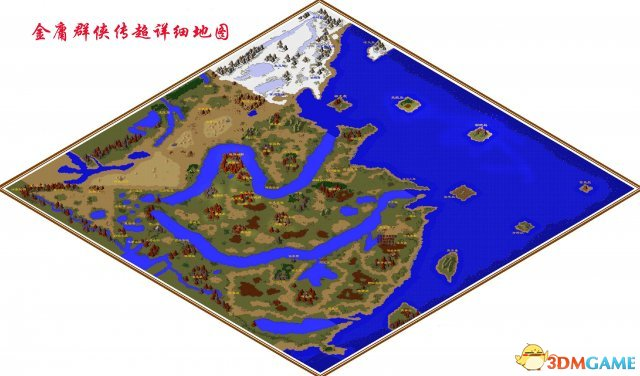# 金庸群侠传游戏地图 金庸群侠传地点坐标

[来源：未知 作者：永春小孩 发表时间：2022-12-03 21:34 阅读次数：]
 《金庸群侠传》是由河洛工作室开发的一款中国武侠RPG游戏，于1996年发行。本作以金庸笔下的十四部武侠小说所改编，玩家可以与部份金庸小说人物共同冒险。游戏内自由度非常高，绝大部分的人物、武功、物品及剧情发展都十分忠于金庸原著。 下面贴出游戏地图，并附上坐标。 地图坐标 白驼山(141,433) 百花谷(160,271) 北丑居(51,109) 冰火岛(102,31) 渤泥岛(274,34) 成昆居(19,249) 程瑛居(295,422) 重阳宫(145,217) 大轮寺(114,297) 峨嵋派(215,408) 福威镖局(369,258) 丐帮(229,256) 高升客栈(198,401) 古墓(144,218) 光明顶(68,397) 海边小屋(286,157) 河洛客栈(359,229) 黑龙潭(309,351) 黑木崖(118,178) 衡山派(355,376) 恒山派(112,215) 洪七公居(169,210) 胡斐居(86,94) 蝴蝶谷(238,219) 华山派(193,302) 回族部落(120,343) 金轮寺(103,397) 绝情谷(133,270) 崆峒派(160,314) 昆仑山(42,420) 昆仑仙境(22,440) 擂鼓山(176,221) 凌霄城(166,404) 灵蛇岛(202,58) 梅庄(332,197) 苗人凤居(223,187) 明教分舵(76,363) 摩天崖(186,338) 南贤居(388,325) 霹雳堂(411,209) 平一指(215,196) 破庙(19,181) 青城派(185,370) 沙漠废墟(53,219) 少林派(247,300) 神龙教(130,100) 思过崖(187,301) 嵩山派(242,293) 泰山派(193,139) 桃花岛(341,138) 天宁寺(330,237) 田伯光居(385,307) 铁掌山(302,343) 万鳄岛(429,136) 无量山(168,426) 无名岛(422,29) 武当派(226,340) 五毒教(247,424) 侠客岛(435,319) 星宿海(132,410) 薛慕华居(192,261)